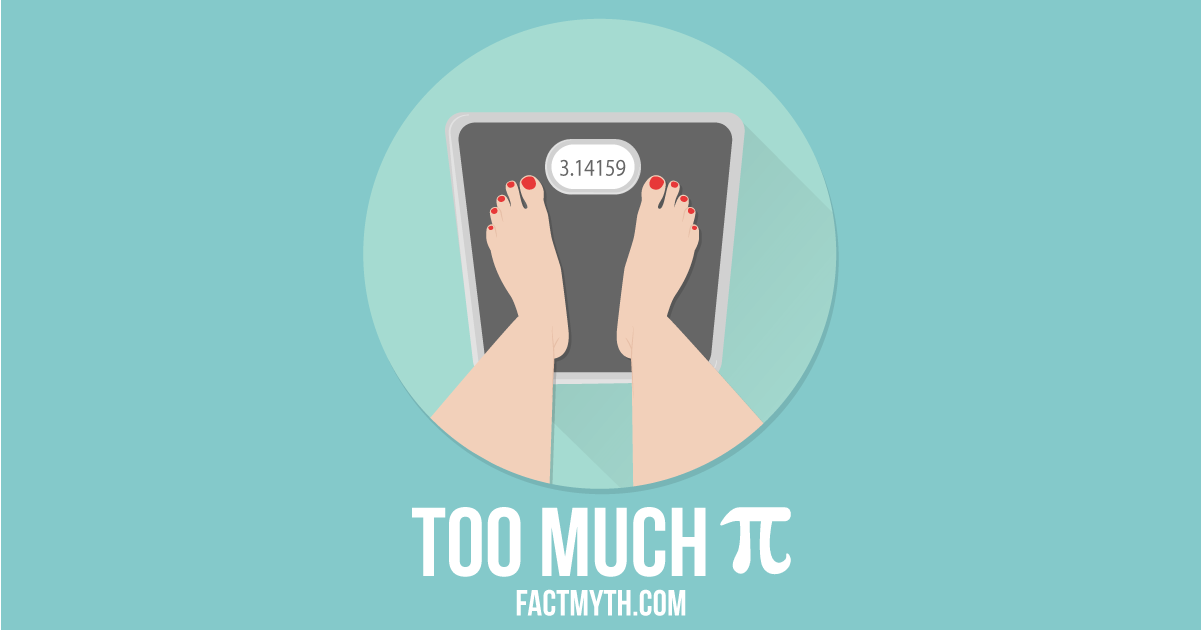Fact

Pi is an irrational number

## What Does it Mean that Pi (π) is an Irrational Number?

Pi (π) is an irrational number, meaning it represents a real number with a non-repeating pattern that can’t fully be expressed. Although Pi has an unrepresentable number of digits in its decimal representation, it can be approximated as 3.14159.

FACT: Pi represents the ratio of a circle’s circumference to its diameter.

## What is an Irrational Number?

An irrational number is any real number that cannot be expressed as a ratio of whole numbers or represented as terminating or repeating decimals. 

A SciShow video on mathematics‘ most delicious irrational number π (although the golden ratio φ also looks rather delicious).

Proof that Pi is Irrational: Johann Heinrich Lambert proved that Pi was irrational in 1761. Proof that Pi is irrational can be found here.

## Is Pi an Infinite Number?

Pi is not an infinite number, it is an irrational number.

• Infinite is a concept that means “can’t be expressed by a real number”.
• Irrational refers to a real number that “can’t be expressed as a fraction and doesn’t repeat a pattern”.

Pi’s decimal representation never settles into a permanent repeating pattern and can’t be fully expressed on paper, so it is infinite in these ways. However, technically speaking, infinity is an abstract concept and not a number.

Given the aforementioned, Pi is not an “infinite number” that “never ends”, it is a real number between 3 and 4, with a non-repeating pattern that cannot be fully expressed as a ratio of integers (i.e. as a fraction).

This is different from something like 1/3 which can be expressed as .333… (a rational number, a repeating pattern, with an infinite number of digits).

Generally, there are many different types of infinity. Both rational and irrational numbers are infinite in that they “can’t be expressed by a real number”, but only irrational numbers “can’t be expressed as a fraction and don’t repeat a pattern”. Since infinity is a concept, and not a number, neither rational or irrational numbers are “infinite numbers”.

## Does Pi “Never End”?

Pi is irrational, as it doesn’t repeat a pattern, but that doesn’t mean we should say “Pi never ends” (for much the same reasons we wouldn’t say Pi is an infinite number). Never ending is not the same as irrational as explained here.

This is just a technicality, but Pi shouldn’t be considered “never ending” or “infinite”, it is simply irrational, meaning that you can’t get a natural number by multiplying Pi by some other natural number.

## What is Pi?

Pi (π) a mathematical constant, that represents the ratio of a circle’s circumference (C) to its diameter (d) expressed as π = C/d. The result of Pi is always the same, approximately 3.14159…## Why is Pi Useful?

Pi is useful because it is an easy to use mathematical constant that can calculate a circle (to varying degrees of accuracy based on how many digits are used). So we can, for instance, find the circumference of a circle by only knowing Pi and the diameter of the circle. Get a more in-depth explainer here.

## Representing Pi in Numbers

We can never write Pi as an exact string of numbers (as the string never ends), so we will always have to approximate. The simplest approximation of Pi used is typically something like 3.14 or 3.1415, although lots of examples use 3.14159. Every decimal we add makes the number more exact. Sometimes Pi is approximated to 3.141592653589, bringing us to the second 9 in the string. You could also use a bunch of “not 101 level” math to represent Pi, like this:

π=Γ(12)2
and
π=6(i=11i2)‾‾‾‾‾‾‾‾‾‾‾√

FACT: π is also a transcendental number. This means it’s a number that is not the root of any normal number (nonzero polynomial having rational coefficients).

## Can We Create a Perfect Circle With Pi?

As far as we know we can create a perfect circle on paper (using an equation like this x^2+y^2=r^2), but we can’t actually create a perfect circle in real life.

TIP: In real life one can measure Pi by constructing a physical wheel and rolling it out, but one won’t get more than a digit or two of accuracy.

We can’t fully write out every digit of Pi, but we can use the first few digits of the number to calculate “good enough” circles and even translate them into notes on a scale (for fun) to play a “Fugue on Pi”.

FACT: In physics there is no such thing as a perfect circle (or a perfect square for that matter). The laws of physics are often about quantizing and getting close enough. See Planck’s constant.

## Has Anyone Ever Tried to Fully Calculate Pi?

We know that we can’t write out Pi fully by hand, or with classic computers, and all signs and proofs point to it being a true irrational number. 

So far Pi has been calculated up to 10 trillion digits. Check out the first million digits of Pi here.

Conclusion

Pi is an irrational number and this has been proved on paper since Johann Heinrich Lambert proved it in 1761 (although Pi was used long before it was proven to be irrational).

References